##### Managerial Economics For Dummies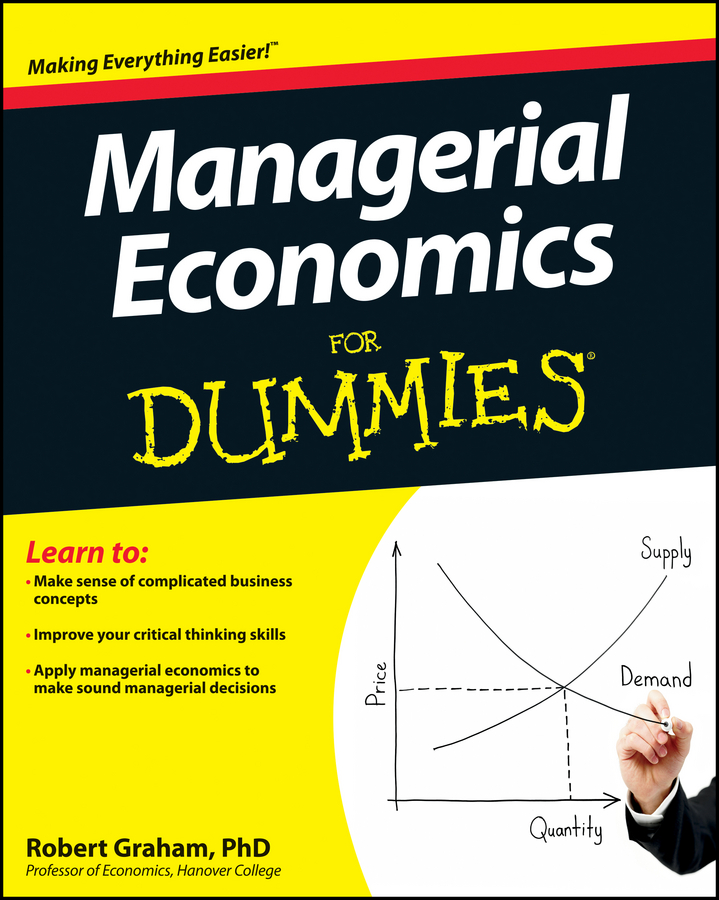Cost-plus pricing means that you determine price by starting with the good’s cost and then adding a fixed percentage or amount to that cost. One of the primary reasons cost-plus pricing is so popular is its simplicity.

Often information on marginal revenue and marginal cost is difficult to obtain with precision, making it impossible to exactly determine the point of profit maximization. By using cost-plus pricing, you can simply include a desired rate of return in the mark-up.

Another advantage of cost-plus pricing is its desirability from the standpoint of public relations. This pricing technique provides an obvious rationale for price increases when cost increases occur.

Cost-plus pricing typically involves two steps. First, the firm determines the per unit cost or average total cost of producing the good. Because average total cost varies as the quantity of output produced changes, the firm’s determination of per unit cost requires the specification of an output level.

After the firm establishes the per unit cost, the firm adds a mark-up to the per unit cost. The mark-up is typically in the form of a percentage, and it represents costs that can’t be easily allocated to a specific product produced by the firm plus a return on the firm’s investment.

The following equation illustrates how to determine price with cost-plus pricing: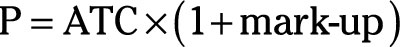where P is the good’s price, ATC is the average total cost or cost per unit, and the mark-up is the percentage added to average total cost.

One criticism of cost-plus pricing is that it focuses on average rather than marginal costs. Because profit maximization requires marginal cost equals marginal revenue, cost-plus pricing may not result in profit maximization.

Another criticism of cost-plus pricing is that it ignores demand conditions. By ignoring demand, the firm can establish a cost-plus price that’s above the market’s equilibrium price, resulting in a surplus. As a consequence, the firm doesn’t sell all the units it produces.

It’s logical to wonder whether cost-plus pricing ever maximizes profit. In order for profit-maximization to occur, cost-plus pricing must result in the firm producing the output level where marginal revenue equals marginal cost.

In the short-run, the difference between marginal cost and average total cost may be sizeable. However, studies have shown that long-run average total cost is typically constant for many firms. Constant long-run average total cost implies constant marginal cost; therefore, marginal cost equals average total cost in this situation. The use of average total cost in the place of marginal cost for pricing results in minimal differences, or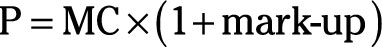Still, because this simple approach ignores demand, it’s unlikely to result in maximum profit. However, when you use marginal cost in the previous equation, it looks very similar to the profit-maximizing equation.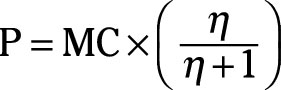Thus, if one plus your mark-up equals the second part of the earlier equation, or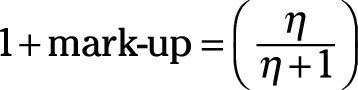Manipulating the earlier equation allows you to determine the mark-up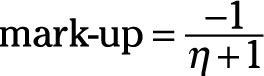Your company determines that the price elasticity of demand for its product is –4. In order to determine the profit-maximizing mark-up, you take the following steps:

1. Substitute –4 for the price elasticity of demand in the mark-up equation.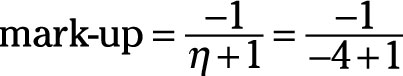2. Calculate the value of the denominator.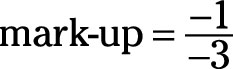3. Divide the numerator by the denominator.

The resulting value is 0.33, or the markup should be 33%.